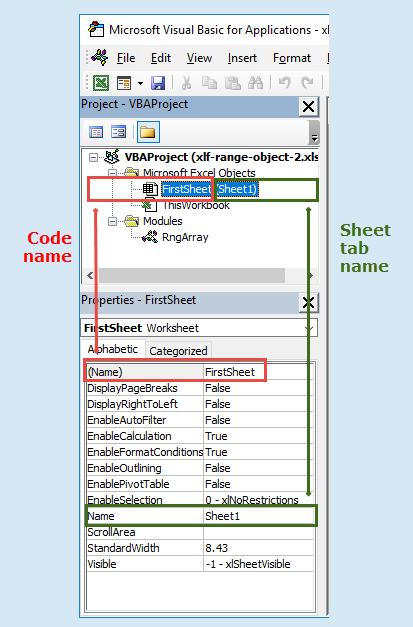# Range object - 1

Object hierarchy:

1. Application (Excel itself)
2. +—— Workbook object, a member of the workbooks collection
3. +—— Worksheet object, a member of the worksheets collection
4. +—— Range object(can be a single cell, multi-cell or non-contiguous). There is no cell object

Examples of Excel object hierarchy, and addressing of the range object with Cells, Item, and Offset properties. Values are written to an array. This allows easy matching of the Immediate Window values with the code statement.

### Preliminary

Code 1: Sub DemoRangeOne preliminary

Sub DemoRangeOne()
' Required:
'   Workbook: xlf-demo-range.xlsm
'   Worksheet: Sheet1
'   Cells:
'        B3: 300
'        B4: 400
'        B5: 500
'
' Name: Data3Row, RefersTo: =$B$3:$B$5

Dim x(1 To 60) As Variant, i As Integer: Const n As Integer = 3


1. Line 12: Declare the x array as type variant. All unpopulated elements of x will be "empty". The colon ":" is a statement concatenation operator, and combines two declaration statements into one line. Declare a constant, n of type integer with value 3

### Application hierarchy

Code 1a: Sub DemoRangeOne with examples of Excel hierarchy

Debug.Print vbNewLine & "==== Print time: " & Time & vbNewLine

x(1) = Application.Workbooks("xlf-vba-range-1.xlsm").Worksheets("Sheet1").Range("B3").Value
x(2) = Workbooks("xlf-vba-range-1.xlsm").Worksheets("Sheet1").Range("B3").Value
x(3) = Worksheets("Sheet1").Range("B3").Value
x(4) = Worksheets(1).Range("B3").Value
x(5) = Range("Sheet1!B3").Value

x(6) = FirstSheet.Range("B3").Value

FirstSheet.Activate


1. Line 25: The worksheet with tab name Sheet1, and codename FirstName is now the active sheet (see figure 1). In the following code, tab Sheet1 is the ActiveSheet. See figure 1 for an explanation of codename.
2.Fig 1: VBE Properties Window - Codename (Name) FirstSheet, tab name Name Sheet1
3. The codename property can only be set in the VBE, and can be used to keep track of the Sheet if the user changes the tab name or tab position

### Single cell

Code 1b: Sub DemoRangeOne with single cell …
x(9) = "==== ActiveSheet has been set": x(10) = "Single cell ..."
x(11) = ActiveSheet.Range("B3").Value
x(13) = Range("B3").Value
x(14) = Cells(3, 2)
x(15) = [B3]


### Multi cell

Code 1c: Sub DemoRangeOne with multi cell …
x(20) = "==== Multi cell ..."
x(21) = Application.Sum(Range("B3:B5"))
x(22) = Application.Sum(Range("Data3Row").Value)
x(23) = Application.Sum([Data3Row])


### .Cells and .Item

Code 1d: Sub DemoRangeOne with .Cells and .Item

x(30) = "==== Cells ..."
x(31) = Range(Cells(3, 2), Cells(3, 2))                 ' <= B3 first cell

x(32) = Application.Sum(Range(Cells(3, 2), Cells(5, 2)))

x(33) = Range("B3").Cells(1, 1)                         ' <= B3 first cell
x(34) = Range("B3").Cells.Item(1, 1)
x(35) = Range("B3").Item(1, 1)
x(36) = Range("B3").Item(1, "A")
x(37) = Range("B3").Item(1)

For i = 1 to n
x(38) = x(38) + Range("B3").Item(i)
Next i

x(40) = Range("B3").Cells(3, 1)                         '  <= B5 last cell
x(41) = Range("B3").Cells(3, "A")
x(42) = Range("B3").Cells.Item(3, 1)
x(43) = Range("B3").Item(3, 1)


Alternate code using a With...End With construct

With Range("B3")
x(33) = .Cells(1, 1)
x(34) = .Cells.Item(1, 1)
x(35) = .Item(1, 1)
x(36) = .Item(1, "A")
x(37) = .Item(1)

For i = 1 to n
x(38) = x(38) + .Item(i)
Next i

x(40) = .Cells(3, 1)                                    '  <= B5 last cell
x(41) = .Cells(3, "A")
x(42) = .Cells.Item(3, 1)
x(43) = .Cells.Item(3)
End With

1. Line 41: The range cells property must include the first and last cell, even when a single cell is addressed. If not x(31) = Range(Cells(3, 2)) returns Method 'Range' of object '_Global' failed. R1C1 references with Range(Application.Indirect("R3C2") cannot be used because the Excel INDIRECT function is not available in VBA
2. Lines 43: Sum the range (R3C2:R5C2) with the WS SUM function
3. Lines 51 to 53: Sum the range using first principles (x = x + y) with a For...Next loop. Add y to the x variable on the right, then assign the sum to the x variable on the left. The Item values are read row by row

### .Offset

Code 1e: Sub DemoRangeOne with .Offset and .Range
x(50) = "==== Offset ..."
x(51) = Range("B3").Offset(0, 0)
x(52) = Range("B3").Offset(2, 0)                        ' <= B5 last cell

x(53) = Range("B3").Range("A1")
x(54) = Range("B3").Range("A3")                         ' <= B5 last cell

For i = 1 To 60
If Not IsEmpty(x(i)) Then Debug.Print i & ". " & x(i)
Next i

End Sub

1. Line 56: The range object is a single cell Range("B3"), and Offset property returns a range of the same dimension. Offset has base 0. Offset(0, 0), zero rows and zero columns, is the range object itself
2. Lines 62 to 64: Right the values from non empty elements of the x array to the Immediate Window. Detect any empty elements with IsEmpty function

Selected VBA items used in this module.

VBA Description
.Cells(RowNumber, ColumnNumber) (property)Returns a range object referencing the cells in a specified range
When used alone, and without a qualifier, Cells refers to all of the cells from the ActiveSheet object. Equivalent to ActiveSheet.Cells
When used as a property to a range object, Cells returns all the cells relative to that object. Use RowNumber, ColumnNumber to reference specific cells
RowNumber is the cell row number
Column is the column, identified by number or alphabetical character
.Items(RowIndex, ColumnIndex) (property)Returns a range object referencing the cells at an offset to the specified range (base 1,1)
RowIndex (required) - read elements in order from left to right, row by row
ColumnIndex (optional) - numeric or alphabet
.Offset(RowOffset, ColOffset) (property)Returns a range object referencing the cells at an offset to the specified range
RowOffset (optional) with default (base) zero . Positive values down, and negative values up
ColOffset (optional) with default (base) zero. Positive values right, and negative values left
Offset inherits the height and width from the Range object

• This example was developed in Excel 2016 Pro 64 bit.
• Revised: Wednesday 20th of September 2017 - 07:41 AM, [Australian Eastern Time (AET)]